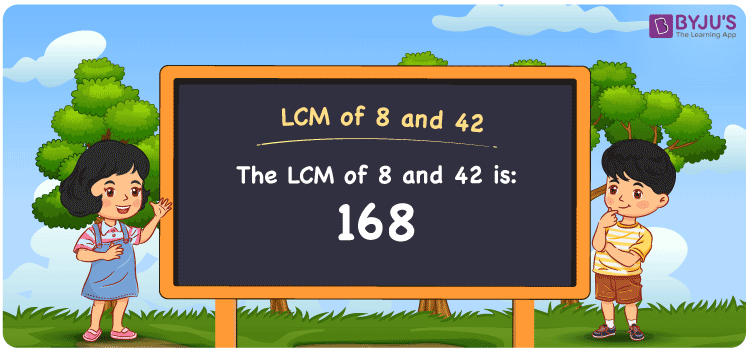# LCM of 8 and 42

LCM of 8 and 42 is 168. LCM which is Least Common multiple or Lowest Common Multiple is the smallest numeral that is divisible by the given set of numbers. Consider the example for finding the LCM of 8 and 42. The answer is 168. 168 is divisible by both 8 and 42. Even 1680 is divisible by 8 and 42, however it is not the LCM for 8 and 42. The smaller number than 1680 is 168 which is divisible by both 8 and 42. Hence 168 is the Least Common Multiple for 8 and 42. You can refer to LCM for more information.

## What is LCM of 8 and 42

The Least Common Multiple or Lowest Common Multiple of 8 and 42 is 168.## How to Find LCM of 8 and 42?

LCM of 8 and 42 can be determined using three methods:

• Prime Factorisation
• Division method
• Listing the multiples

### LCM of 8 and 42 Using Prime Factorisation Method

We can write 8 and 42 as the product of prime numbers, such that;

8 = 2 × 2 × 2

42 = 2 × 3 × 7

Multiplying 2, which is the common factor of both with other prime factors of both 8 and 42, forms the LCM.

LCM (8, 42) = 2 × 2 × 2 × 3 × 7 = 168

### LCM of 8 and 42 Using Division Method

In the Division Method, the numbers 8 and 42 are divided together by prime divisors. These are multiplied to get the LCM.

 2 8 42 2 4 21 2 2 21 3 1 21 7 1 7 × 1 1

LCM (8, 42) = 2 × 2 × 2 × 3 × 7 = 168

### LCM of 8 and 42 Using Listing the Multiples

By listing all the multiples of 8 and 42, we can identify the LCM. Below is the list of multiples for 8 and 42

Multiples of 8

8, 16, 24, 32, 40, 48, 56, 64, 72, 80, 88, 96, 104, 112, 120, 128, 136, 144, 152, 160, 168, 176, 184

Multiples of 42

42, 84, 126, 168, 210, 252

LCM (8, 42) = 168

## Video Lesson on Applications of LCM## Solved Examples

What is the smallest number that is divisible by both 8 and 42?

Answer: 168 is the smallest number that is divisible by both 8 and 42.

What is the LCM of 2, 4, 8 and 48?

Answer: 168 is the LCM of 2, 4, 8 and 48 as 2 and 4 are the factors of 4 and 48.

## Frequently Asked Questions on LCM of 8 and 42

### What is the LCM of 8 and 42?

The LCM of 8 and 42 is 168.

### Are LCM of 8 and 42 same as the LCM of 4 and 8?

No. LCM of 8 and 42 is 168 and LCM of 4 and 8 is 8. They are not the same.

### Are Least Common Multiple and Least Common Divisor both the same for 8 and 42?

Yes. The LCM (Least common multiple) and LCD (Least Common Divisor) are both the same for 8 and 42  which is 168.

### What are the first 3 common multiples of 8 and 42?

The first three common multiples of 8 and 42 are 168, 336, and 504.

### Is the LCM of 4 and 8 the same as the LCM of 6 and 42?

No. LCM of 4 and 8 is 8, whereas the LCM of 6 and 42 is 42. They are not the same.